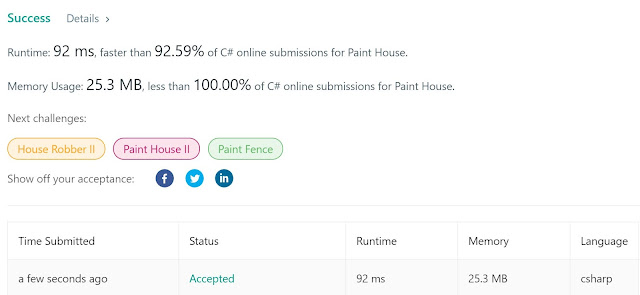## Posts

Showing posts from April, 2020

### Adding one row to a Binary TreeLove tree problems, once you get it right, there are usually very few corner cases (other than the d=1, which was mentioned in the problem description anyways). Here it is, and code is down below. Song that goes along well with this problem:  https://www.youtube.com/watch?v=7HHBFkdHyRU&list=PL9LM05lDUoXqVDYM5MIM4owPiYLCm_Ucm&index=385 Cheers, ACC. https://leetcode.com/problems/add-one-row-to-tree/ 623. Add One Row to Tree Medium 378 119 Add to List Share Given the root of a binary tree, then value  v  and depth  d , you need to add a row of nodes with value  v  at the given depth  d . The root node is at depth 1. The adding rule is: given a positive integer depth  d , for each NOT null tree nodes  N  in depth  d-1 , create two tree nodes with value  v  as  N's  left subtree root and right subtree root. And  N's   original left subtree  should be the left subtree of the new left subtree root, its  original right subtree  should be the right subtree o

### Covid and Fibonacci Source Code

public class CovidAndFibonacci {     public static void CovidAndFibonacciApproximation()     {         DateTime ed = new DateTime(2020, 4, 18);         double target = 2330793;         double best = 0;         double bestActualVal = 0;         double bestx = 0;         double besty = 0;         for (double x = 0.1; x < 1; x += 0.1)         {             for (double y = 0.1; y < 1; y += 0.1)             {                 //Fibo                 double f1 = 0;                 double f2 = 1;                 DateTime dt = new DateTime(2020, 1, 1);                 while (DateTime.Compare(dt, ed) <= 0)                 {                     double temp = f2;                     f2 = f1 * x + f2 * y;                     f1 = temp;                     dt = dt.AddDays(1);                 }                 if (best == 0 || Math.Abs(target - f2) < best)                 {                     bestActualVal = f2;                     best = Math.Abs(target - f2)

### Minimum Number of Fibonacci NumbersInteresting problem, fresh out of the oven:  https://leetcode.com/problems/find-the-minimum-number-of-fibonacci-numbers-whose-sum-is-k/ 1414. Find the Minimum Number of Fibonacci Numbers Whose Sum Is K Medium 26 5 Add to List Share Given the number  k ,  return the minimum number of Fibonacci numbers whose sum is equal to  k , whether a Fibonacci number could be used multiple times. The Fibonacci numbers are defined as: F 1  = 1 F 2  = 1 F n  = F n-1  + F n-2  , for n > 2. It is guaranteed that for the given constraints we can always find such fibonacci numbers that sum  k . Example 1: Input: k = 7 Output: 2 Explanation: The Fibonacci numbers are: 1, 1, 2, 3, 5, 8, 13, ... For k = 7 we can use 2 + 5 = 7. Example 2: Input: k = 10 Output: 2 Explanation: For k = 10 we can use 2 + 8 = 10. Example 3: Input: k = 19 Output: 3 Explanation: For k = 19 we can use 1 + 5 + 13 = 19. Constraints: 1 <= k <= 10^9 Accepted 4,104 S

### The Power of C# List ClassThe solution to this problem demonstrates the power of the C# List Class . Here it is:  https://leetcode.com/problems/queries-on-a-permutation-with-key/ 1409. Queries on a Permutation With Key Medium 20 40 Add to List Share Given the array  queries  of positive integers between  1  and  m , you have to process all  queries[i]  (from  i=0  to  i=queries.length-1 ) according to the following rules: In the beginning, you have the permutation  P=[1,2,3,...,m] . For the current  i , find the position of  queries[i]  in the permutation  P  ( indexing from 0 ) and then move this at the beginning of the permutation  P.  Notice that the position of  queries[i]  in  P  is the result for  queries[i] . Return an array containing the result for the given  queries . Example 1: Input: queries = [3,1,2,1], m = 5 Output: [2,1,2,1] Explanation: The queries are processed as follow: For i=0: queries[i]=3, P=[1,2,3,4,5], position of 3 in P is 2 , then we move 3 to the beginning